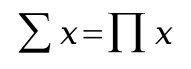## Sum equals productI
love investigating patterns in numbers and shapes, I particularly like finding equations describing the patterns. Few years ago, while working on a maths assignment, I wondered if the number 2 is the only number that gives the same answer when added together and multiplied together i.e 2+2=2*2=4. It seemed obvious that 2 had to be the only number that you can do this with, but was there other numbers that when added together N number of times would give the same result as being multiplied together N times. So I decided to investigate and see where it leads me. I originally called the results of these sums and products 'perfect numbers P' , but I later realised that perfect number was a name given to something else.
I'll start with defining what the number P is, P satisfies the following:Where N is the number of terms and x is the number we're adding and multiplying together. If we equate the above two and rearrange to get:From equations 3 and 4 we can immediately see that N cannot equal zero or one otherwise we get undefined results. I'll show you later how N can approach 0 and 1 to give some nice results but first I want to use equations 3 and 4 to produce some values of x and P.Dx and Dp are the difference in the values of x and P respectively. From the table we can see that Dp seems to be converging to 1 and Dx is converging towards 0. This tells us that as N goes to infinity so does P, x however is converging towards a single value of 1.
Now lets consider what happens when N=0. We already know that we can't just enter 0 into the equation, but we can sort of cheat and say what happens when N=0+h where h can be as small as we like, or to put it another way the lim(0+h)=0 as h approaches 0. If you can remember your basic differentiation you'll know what I have done there. So if we put this new value into equation 4 we get P=1. A far more interesting result is obtained when we apply the same method to N=1. So once again the lim(1+h) = 1 as h approaches 0. Now if you put that into equation 4 we get p=e where e is the base of the natural logarithm also known as Euler's number. I don't know about you but I find this result amazing. We can also do the same thing for x. When N=0+h we get x=infinity and when N=1+h we once again get the number e. One final bit of observation from the results is that P starts from 1 and goes to infinity whereas x starts from infinity and goes to 1.
I realise that this is not very mathematically rigorous but I hope you can appreciate the results. I have not worked on this any further so if anyone else finds any more interesting results please let me know.

Link:
Mathcentral

#### 3 comments:

1.Hi Mohammed, I think perfect numbers (and Marsenne Primes) are an interesting feature of the landscape of math. I've been interested in them for over 20 years. The first time I noticed the pattern was when I was idly looking at the first few perfect numbers, then converting them to binary using my calculator. It was then that I saw a pattern that made me sit up straight.

In case you didn't already know about it, check out the Great Internet Marsenne Prime Search. You can use the spare clock cycles of your computer to work on finding Marsenne primes, just like SETI@home and Folding@home does.

I found my way to your blog via the comment you left on mine. :-)

2.Hi Mohammad,

I've been exploring the "sum equals product" for a while too. You're absolutely right, it is amazing.

Here's another interesting thing.

Let's see the following equation:

x+y=n; x*y=n

If, for example, n=5, we get:

3,618 + 1,382 = 5
3,618 * 1,382 = 5 (approx.)

What about x-y = s ?

3,618 - 1,382 = s

s= 2,236

s = square root of 5 (!)

But it's not applicable for every n; however, something else is:

if x+y=x*y=n,

x-y = sqrt (n * (n-4))

Please send me an e-mail, I would like to share and exchange the experiences.

Best regards,

Moris Mateljan

morismateljan @ gmail.com

3.http://www.amazon.com/b?_encoding=UTF8&site-redirect=&node=165793011&tag=tabbooingcom-20&linkCode=ur2&camp=1789&creative=9325